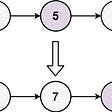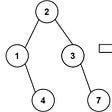# Dijkstra’s Algorithm:

In a weighted graph, the shortest path is the one where the sum of the edges is the smallest. Dijkstra’s Algorithm is an algorithm that solves the Shortest Path Problem in a weighted graph. The shortest path in an unweighted graph is the one with the fewest number of edges. You can simply find it by doing BFS on the graph, and count the number of edges you encounter.

## How does it work:

You start by defining the distance (for each vertex), the sun of edges weights on a path between our starting point and end vertex. The distance value we start is infinity and zero for our starting point. We use a min priority queue to choose every run the smallest distance node, by the smallest weighted edge. Here is an example, This algorithm complexity is O(v²), but can be more efficient if using min-heap to implement a priority queue.

## Knapsack Problem (0–1):

You have a theoretical knapsack with a limited weight capacity. You have items with weight and value. The question is how can I optimize the total value of items in my knapsack. A zero-one, knapsack problem, means that you have a set of items, and you must take or leave a whole object. The solution idea is to find the max value for the min weight and add them up. We use a zero-initialized array to store the maximum possible value for every weight up into our weight limit. We start by taking the first object and check if it is the maximum value from the current value stored in the index of the weight -1. If it is then we can update all the indexes from the weight -1 till the array’s end with the new value. We continue on till we finish checking all our objects. Then we go through every object and check if it can increase the maximum value of every possible weight. Thus, the runtime is O(nW).

## Dynamic Programming:

The idea of dynamic programming is to take a complicated problem and break it down into subproblems. In dynamic programming, you start by finding and solving the base case problem. Then store the base solutions on a lookup table. These are the basics of Dynamic Programming, solving the problem for a trivial case and storing the solution in a lookup table. A problem is a dynamic problem when you can break the base problem into subproblems.

## Traveling Salesman Problem — TSP:

Envision a graph where all the nodes are cities and all the edges are roads between them. What's the fastest route to travel between every city and return home. The traveling salesman problem is an NP-hard problem. NP-hard problems are problems that can't be solved in polynomial time. For these problems, there are two types of solutions. Firstly, Exact Answers. These solutions give the solution, but don’t happen in polynomial time. Secondly, Approximation Algorithms, which do not always find the best solution but give the general near-optimal solution.

--

--

--

## More from Reem Mikulsky

I’m a 17 years old boy that is interested in the future and technology

Love podcasts or audiobooks? Learn on the go with our new app.

## Alexa Skill with Azure Function## Waterfall and agile don’t mix, right?## Build, Test, and Deploy a Flask Application — Part 2## ​​​🚀 Airdrop: ComingChat## Venvs & Pyenvs & Pipenvs, OH MY!## Reem Mikulsky

I’m a 17 years old boy that is interested in the future and technology

## Leetcode#382. Linked List Random Node## LeetCode — Odd Even Linked List## LeetCode 617- Merge Two Binary Trees